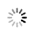# 用java写一段判断数据序列稳定性的代码

2月前 ⋅ 185 阅读
```以下是用Java编写判断数据序列稳定性的代码：

```
public static boolean isStable(int[] arr) {
// 创建一个二维数组，第一维存放数值，第二维存放下标
int[][] pairs = new int[arr.length];

// 初始化二维数组
for (int i = 0; i < arr.length; i++) {
pairs[i] = arr[i];
pairs[i] = i;
}

// 对数值进行排序，使用冒泡排序
for (int i = 0; i < pairs.length; i++) {
for (int j = i + 1; j < pairs.length; j++) {
if (pairs[i] > pairs[j]) {
int tempValue = pairs[i];
int tempIndex = pairs[i];
pairs[i] = pairs[j];
pairs[i] = pairs[j];
pairs[j] = tempValue;
pairs[j] = tempIndex;
}
}
}

// 判断排序后的数组是否稳定
for (int i = 0; i < pairs.length - 1; i++) {
if (pairs[i] == pairs[i + 1] && pairs[i] > pairs[i + 1]) {
return false;
}
}

return true;
}
```

### 相关推荐### 热门帖子### 最新帖子### 最新评论